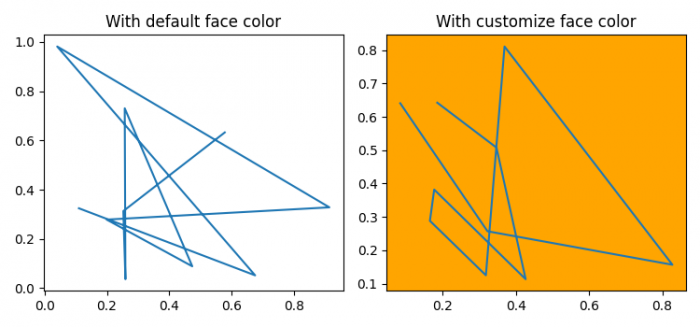# Change the default background color for Matplotlib plots

To change the default background color for Matplotlib plots, we can take the following steps −

## Steps

• Set the figure size and adjust the padding between and around the subplots.
• Get the current axis.
• Add a subplot to the current figure, with nrows=1, ncols=2 and index=1.
• Plot random x and y data points using plots() method.
• Set the title of the subplot.
• Add a subplot to the current figure with nrows=1, ncols=2 and index=2.
• Get the current axis.
• Set the customize face color.
• Plot x and y data points using plot() method.
• Set the title of the plot.
• To display the figure, use show() method.

## Example

import numpy as np
import matplotlib.pyplot as plt

plt.rcParams["figure.figsize"] = [7.50, 3.50]
plt.rcParams["figure.autolayout"] = True

ax = plt.gca()

print("Default face color is: ", ax.get_facecolor())

plt.subplot(121)
plt.plot(np.random.rand(10), np.random.rand(10))
plt.title("With default face color")
plt.subplot(122)

ax = plt.gca()
ax.set_facecolor("orange")

plt.plot(np.random.rand(10), np.random.rand(10))
plt.title("With customize face color")

plt.show()

## Output